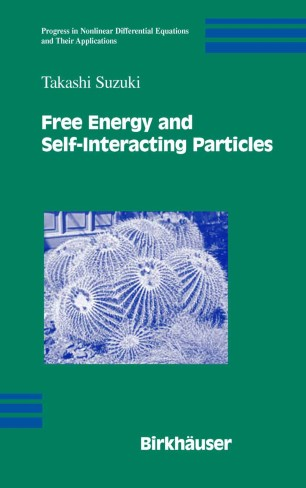# Free Energy and Self-Interacting Particles

• Takashi SuzukiBook

Part of the Progress in Nonlinear Differential Equations and Their Applications book series (PNLDE, volume 62)

1. Front Matter
Pages i-xiii
2. Pages 1-23
3. Pages 25-34
4. Pages 35-58
5. Pages 59-77
6. Pages 79-103
7. H Tanaka
Pages 105-113
8. Pages 115-145
9. Pages 147-173
10. Pages 175-205
11. Pages 207-218
12. Pages 219-245
13. Pages 247-275
14. Pages 277-291
15. Pages 293-306
16. Pages 307-322
17. Pages 323-343
18. Back Matter
Pages 345-366

### Introduction

This book examines a system of parabolic-elliptic partial differential eq- tions proposed in mathematical biology, statistical mechanics, and chemical kinetics. In the context of biology, this system of equations describes the chemotactic feature of cellular slime molds and also the capillary formation of blood vessels in angiogenesis. There are several methods to derive this system. One is the biased random walk of the individual, and another is the reinforced random walk of one particle modelled on the cellular automaton. In the context of statistical mechanics or chemical kinetics, this system of equations describes the motion of a mean ?eld of many particles, interacting under the gravitational inner force or the chemical reaction, and therefore this system is af?liated with a hierarchy of equations: Langevin, Fokker–Planck, Liouville–Gel’fand, and the gradient ?ow. All of the equations are subject to the second law of thermodynamics — the decrease of free energy. The mat- matical principle of this hierarchy, on the other hand, is referred to as the qu- tized blowup mechanism; the blowup solution of our system develops delta function singularities with the quantized mass.

### Keywords

Applied Mathematics Green's function Mathematical Biology Mathematical Physics Partial Differential Equations STATISTICA calculus differential equation mechanics modeling partial differential equation quantum mechanics thermodynamics

### Editors and affiliations

• Takashi Suzuki
• 1
1. 1.Department of System Innovation Division of Mathematical ScienceOsaka University Graduate School of Engineering ScienceOsakaJapan